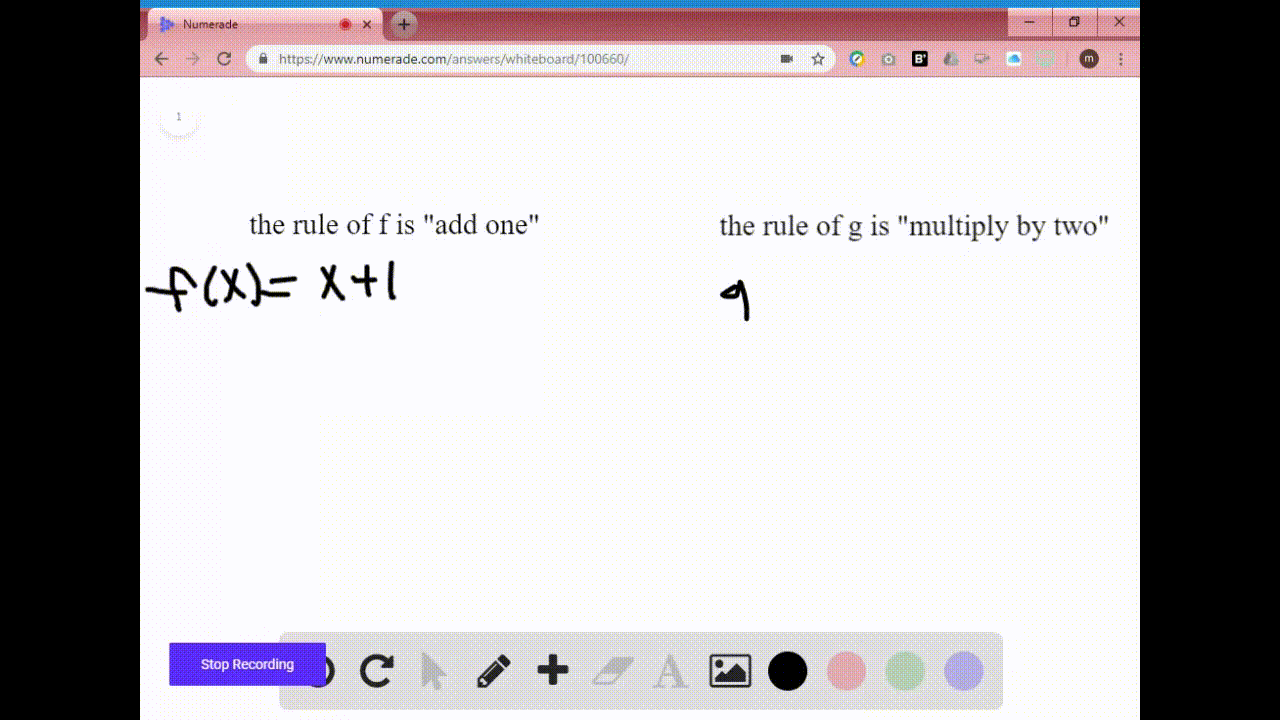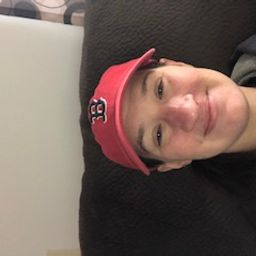Enroll in one of our FREE online STEM summer camps. Space is limited so join now!View Summer Courses### We can express the functions in Exercise 3 algebr…

02:03Syracuse University

Need more help? Fill out this quick form to get professional live tutoring.

Get live tutoring
Problem 3

If the rule of the function $f$ is "add one" and the rule of the function $g$ is "multiply by $2, "$ then the rule of $f \circ g$ is "___________" and the rule of $g \circ f$ is "___________"

The rule of $f_{o} g$ is multiply by two then add one
and the rule of $g_{o} f$ is add one then multiply by two

## Discussion

You must be signed in to discuss.

## Video Transcript

in this problem for being asked to fill in the blanks with the correct phrase. While we're told that the rule for the function F is to add one, a number told the rule for their function. Fergie is the multiply too. So now we want to find a rule for F G. Well, that means the composition. So remember this really means f of G of X. And if you remember how compositions work, that means we have to start with the inside. So first, what we would have to dio is multiply by two. Then we would add one. So remember, with compositions, you always do the inside first. So now for the second plane, we want to do G compass position with F, so that would be the opposite. We would first start with F, which is to add one. Then we would have to multiply by two